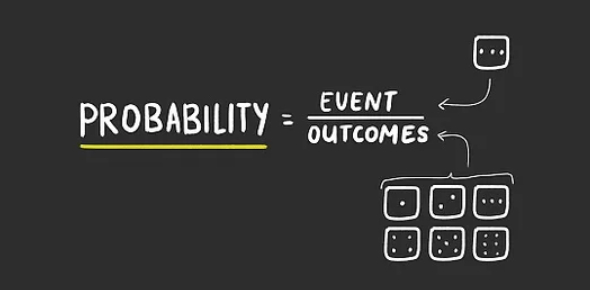# Probability - Multiple Choice

10 Questions | Total Attempts: 13189SettingsProbability is a measure of the likelihood that an event will occur. The higher the probability of an event, the more likely it is to occur, i. E. Tossing a coin gives a 50% chance of getting heads or tails.

• 1.
If you rolled a 6-sided dice, what is the probability of rolling a 3?
• A.

1/6

• B.

2/3

• C.

3/6

• D.

5/6

• 2.
If you flipped 2 coins, what is the probability that both will land on tails?
• A.

2/4

• B.

3/4

• C.

1/4

• D.

0/4

• 3.
If you rolled a 6-sided dice, what is the probability of rolling a even number?
• A.

2/6

• B.

3/6

• C.

4/6

• D.

5/6

• 4.
A lolly bag contains 2 red, 3 green and 2 blue gum balls. What is the probability of selecting a green one?
• A.

2/7

• B.

5/7

• C.

4/7

• D.

3/7

• 5.
A card is selected from a deck of playing cards. What is the probability of selecting a red card?
• A.

1/4

• B.

3/4

• C.

0/2

• D.

1/2

• 6.
What is the probability of selecting the diamond suit from a deck of playing cards?
• A.

1/4

• B.

2/4

• C.

3/4

• D.

0/4

• 7.
What is the probability of rock beating paper?
• A.

0/3

• B.

1/3

• C.

2/3

• D.

1

• 8.
If you flipped a coin, what is the probability it will land on heads?
• A.

0/2

• B.

1/4

• C.

3/4

• D.

1/2

• 9.
There are red, yellow and green lollipops in a bag. What is the probability of selecting a blue one?
• A.

1/4

• B.

1

• C.

0/3

• D.

2/3

• 10.
What is the probability of paper losing to scissors?
• A.

0/3

• B.

1/3

• C.

2/3

• D.

1

Related TopicsBack to top